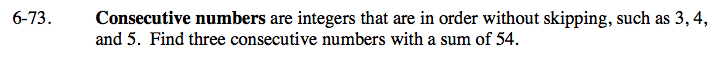### Home > CAAC > Chapter 6 > Lesson 6.2.4 > Problem6-73

6-73.

Consecutive numbers are integers that are in order without skipping, such as 3, 4, and 5. Find three consecutive numbers with a sum of 54. Homework Help ✎If x is the first integer, then x + 1 would be the second. What would be the third integer?